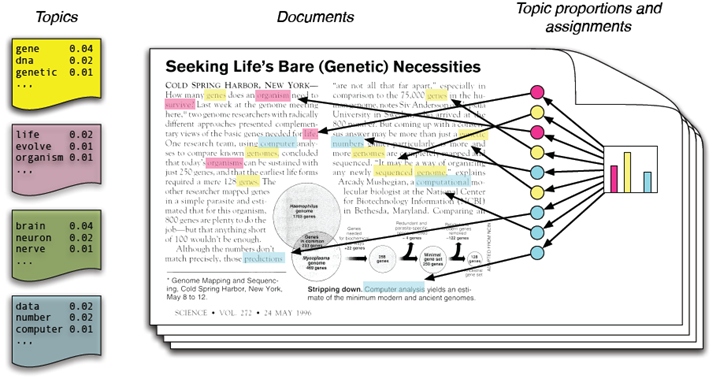# 信息的度量：信息熵的理解与应用

## 什么是信息熵？$$H(X)=-\sum_{x\in\chi}p(x)\log{p(x)}$$

• 单调性，发生概率越高的事件，其携带的信息量越低；
• 非负性，信息熵可以看作为一种广度量，非负性是一种合理的必然；
• 累加性，即多随机事件同时发生存在的总不确定性的量度是可以表示为各事件不确定性的量度的和，这也是广度量的一种体现。

• 假设存在一个随机变量$x$，当我们观测到该随机变量的一个样本时，我们可以接受到多少信息量呢？毫无疑问，当我们被告知一个极不可能发生的事情发生了，那我们就接收到了更多的信息；而当我们观测到一个非常常见的事情发生了，那么我们就接收到了相对较少的信息量。因此信息的量度应该依赖于概率分布$p(x)$，所以说熵h(x)的定义应该是概率的单调函数。
• 假设两个随机变量$x$和$y$是相互独立的，那么分别观测两个变量得到的信息量应该和同时观测两个变量的信息量是相同的，即：$h(x+y)=h(x)+h(y)$。而从概率上来讲，两个独立随机变量就意味着$p(x,y)=p(x)p(y)$，而唯一能够满足由乘法公式变为加法公式的函数即为log函数，所以此处可以得出结论熵的定义$h$应该是概率$p(x)$的log函数。因此一个随机变量的熵可以使用如下定义：$h(x)=-\log{p(x)}$。此处的负号仅仅是用来保证熵（即信息量）是正数或者为零。而log函数基的选择是任意的（信息论中基常常选择为2，因此信息的单位为比特bits；而机器学习中基常常选择为自然常数，因此单位常常被称为nats）。
• 最后，我们用熵来评价整个随机变量$x$平均的信息量，而平均最好的量度就是随机变量的期望，即熵的定义如下：$$H(X)=-\sum_{x\in\chi}p(x)\log{p(x)}$$

• 事件A：巴西队进入了2018世界杯决赛圈。
• 事件B：中国队进入了2018世界杯决赛圈。

• 信息量和事件发生的概率相关，事件发生的概率越低，传递的信息量越大
• 信息量应当是非负的，必然发生的事件的信息量为零（必然事件是必然发生的，所以没有信息量。几乎不可能事件一旦发生，具有近乎无穷大的信息量。）
• 两个事件的信息量可以相加，并且两个独立事件的联合信息量应该是他们各自信息量的和

• 干货：观点独特，有实用价值的信息
• 水货：人人都明白，放着四海皆准的大道理

## 使用信息熵求解问题

1000桶水，其中一桶有毒，猪喝毒水后会在15分钟内死去，想用一个小时找到这桶毒水，至少需要几头猪？

• 一滴毒水足以导致一头猪的死亡。死亡时间为15分钟内不确定的某个时间点。
• 其死亡只是毒水导致的，不会有其他因素导致死亡。
• 猪的承水量无穷大，且假设饮一桶花费时间为零。

• 在1000桶水中找一桶水，需要的信息量是$-\log(\frac{1}{1000})$
• 而一头猪所能提供的信息是它在什么时间死（未死、0-15分钟、15-30分钟、30-45分钟、45-60分钟），因此能提供的信息量是$-\log(\frac{1}{5})$
• 求$-\log(\frac{1}{1000}) \leq -n\log(\frac{1}{5})$可得，n>4.29203即，5头猪即可。

• 将1000桶水按5进制编号，将五头猪对应到每一位。
• 首先喂每头猪5进制编号下该位数为0的水。15分钟内，如果某头猪死了，那么有毒的水该位就是0；
• 然后过15分钟后，再喂还存活的猪5进制编号下该位数为1的水。15-30分钟内，如果某头猪死了，那么有毒的水该位就是1。
• 以此类推，于是在一个小时内我们就可以判断有毒的水的编号在5进制下每一位是多少，从而找到这桶水。

## 数据压缩与信息熵

• 第一类压缩过程是可逆的，也就是说，从压缩后能够完全恢复，信息没有任何丢失，称为无损压缩
• 第二类压缩过程是不可逆的，无法完全恢复，信息有一定的丢失，称为有损压缩。

• 第一步是得到文件内容的概率分布，哪些部分出现的次数多，哪些部分出现的次数少
• 第二步是对文件进行编码，用较短的符号替代那些重复出现的部分

$$\log_2(\frac{1}{p_1}) + \log_2(\frac{1}{p_2}) + … + \log_2(\frac{1}{p_n}) = \sum \log_2(\frac{1}{p_n})$$

### 霍夫曼编码

 字母 频率 编码 space 7 111 a 4 010 e 4 000 f 3 1101 h 2 1010 i 2 1000 m 2 0111 n 2 0010 s 2 1011 t 2 0110 l 1 11001 o 1 00110 p 1 10011 r 1 11000 u 1 00111 x 1 10010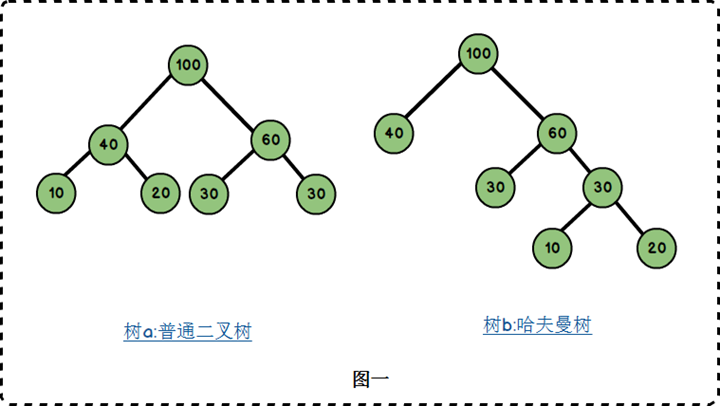• 路径与路径长度：从树中一个节点到另一个节点之间的分支构成了两个节点之间的路径，路径上的分支数目称作路径长度。若规定根节点位于第一层，则根节点到第H层的节点的路径长度为H-1.如树b：100到60 的路径长度为1；100到30的路径长度为2；100到20的路径长度为3。
• 树的路径长度：从根节点到每一节点的路径长度之和。树a的路径长度为1+1+2+2+2+2 = 10；树b的路径长度为1+1+2+2+3+3 = 12.
• 节点的权：将树中的节点赋予一个某种含义的数值作为该节点的权值，该值称为节点的权；
• 带权路径长度：从根节点到某个节点之间的路径长度与该节点的权的乘积。例如树b中，节点10的路径长度为3,它的带权路径长度为10 * 3 = 30；
• 树的带权路径长度：树的带权路径长度为所有叶子节点的带权路径长度之和，称为WPL。树a的WPL = 2 *（10+20+30+40） = 200 ;树b的WPL = 1×40+2×30+3×10+3×20 = 190.而哈夫曼树就是树的带权路径最小的二叉树。

## 通信与信息熵

$$C = B \log_2(1+\frac{S}{N})$$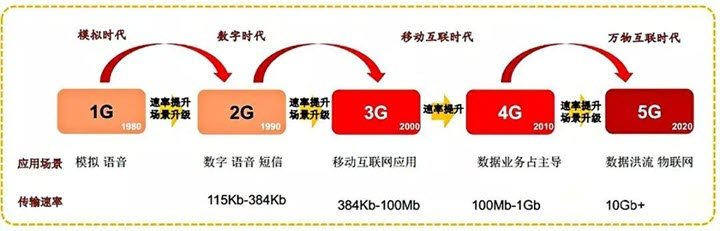### 华为5G与极化码

2008年在国际信息论ISIT会议上，土耳其毕尔肯大学埃达尔·阿利坎（Erdal Arıkan）教授首次提出了这个信道极化的概念，基于该理论，他给出了人类已知的第一种能够被严格证明达到信道容量的信道编码方法，并命名为极化码。

## 信息熵在机器学习的应用

### 信息增益

• 当infoGain > 0，表明集合信息熵减小，包含的信息更纯更有序，价值得到提高。
• 当infoGain < 0，信息变得混沌。
• 当infoGain = 0，信息量没有变化，但不表明信息没有变化。

$$H(X|Y) = \sum_{y \in Y}{p(y) H(X|Y=y)}$$

$$Gain(D,a) = H(D) – H(D|a) = H(D)-\sum_{v=1}^{V}\frac{\left |D^v \right |}{\left |D \right |}H(D^v)$$

$$Gain\_ratio(D,a) = \frac{Gain(D,a)}{IV(a)}$$

$$IV(a) = -\sum_{V}^{v=1} \frac{\left | D^v \right |}{\left |D \right |} \log2 \frac{\left | D^v \right |}{\left |D \right |}$$

### 基尼指数

$$Gini(D)=\sum_{k=1}^{K} p_k(1-p_k)=1-\sum_{k=1}^{K}p_k^2$$

$$Gini(D)=1 – \sum_{k=1}^{K}(\frac{|C_k|}{D})^2$$

$$Gini\_index(D,a)=\sum \limits_{v=1} ^V\frac{\left| D^v \right|}{\left| D \right|}Gini(D^v)$$

$$Gini\_Gini(D,a)=\frac{D_1}{D}Gini(D_1) + \frac{D_2}{D}Gini(D_2)$$

$$a_*=\arg\limits_{a\in A} \min Gini\_index(D,a)$$

• 节点中的样本个数小于预定阈值，
• 样本集的Gini系数小于预定阈值（此时样本基本属于同一类），
• 或没有更多特征。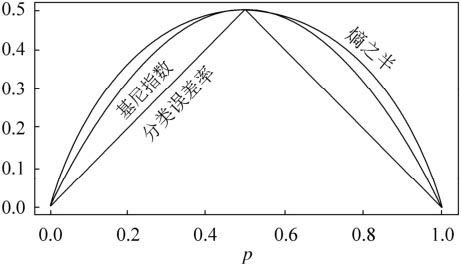### WOE与IV

$$WOE_i = ln(\frac{Bad_i}{Bad_T}/\frac{Good_i}{Good_T}) = ln(\frac{Bad_i}{Bad_T})-ln(\frac{Good_i}{Good_T})$$

IV（Information Value）是与WOE密切相关的一个指标，常用来评估变量的预测能力。因而可用来快速筛选变量。

IV的计算公式定义如下，其可认为是WOE的加权和：

$$IV_i = (\frac{Bad_i}{Bad_T}-\frac{Good_i}{Good_T}) * WOE_i$$

$$IV = \sum_{i=1}^{n}IV_i$$

## 信息熵在营销场景的应用思考

• 营销场景的不一致，导致有的营销转化高，有的营销转化低，不应等同看待
• 划分定义拍脑袋，针对某一个用户，营销5次没有反馈是从首次到第5次，还是5次计算可以间断，还是不需要从首次开始，只要连续5次都算。各种不同的定义无法说清楚那种更好。

• 营销成功，则获得的信息为：$H_s = -\log{R_{conversion}}$，其中$R_{conversion}$为营销计划的转化率
• 营销失败，则获得的信息为：$H_f = -\log{(1-R_{conversion})}$

• 假设转化率为百分之2，则可提供的信息比为：$\frac{-\log\frac{2}{100}}{-\log\frac{98}{100}} \approx 193.64$
• 假设转化率为千分之2，则可提供的信息比为：$\frac{-\log\frac{2}{1000}}{-\log\frac{998}{1000}} \approx 3104.30$

$H(\theta ) < H(F) = \sum_{i=1}^{n}H_{f_n}$

$$H(\theta ) < {H(F)}’ = \sum_{i=1}^{n}(H_{f_n}\cdot 0.99^{d_n})$$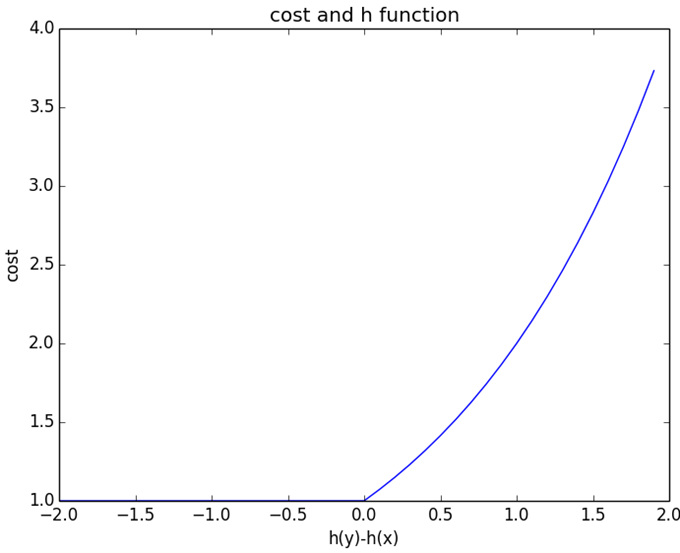##### Learning to Rank算法学习之GBRank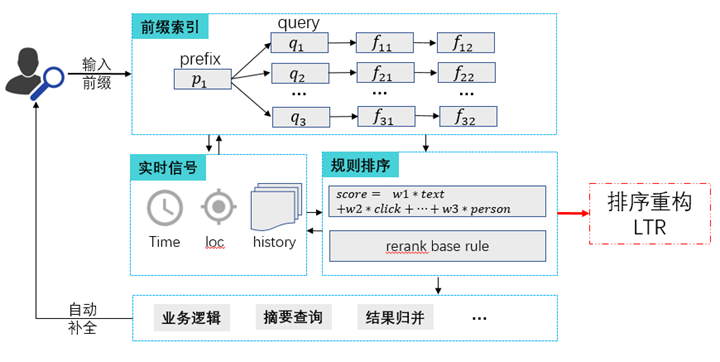##### 推荐系统：从千人千面到千域千面